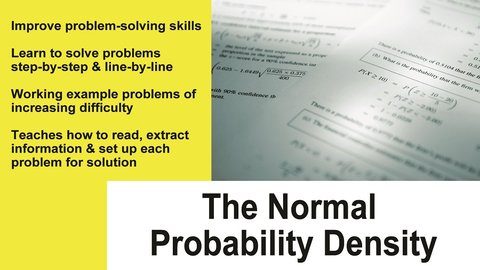# Normal Probability Density Part of the Series: Probability and Statistics Tutor Series

##### TMW Media## Related videos

Probability
Expressing a probability between certainty and impossible as the likelihood of an uncertain event occurring --conducting and recording probability experiments using small and large number trials with replacement --calculating the theoretical probability of a particular event occurring --expressing probabilities as odds
Probability and Statistics Tutor Series
The Probability and Statistics Tutor is a complete 14 lesson series covering all of the core topics in detail. What sets this series apart from other teaching tools is that the concepts are taught entirely through step-by-step example problems of increasing difficulty. It works by introducing each new concept in…
Fundamentals of Probability
This program covers the important topic the fundamentals of probability in Probability and Statistics. We begin by discussing what the concept of probability really means and why it is important. Next, we solve several problems that involve the essential ideas of probability to give students practice with the material.
This program covers the important topic of the addition rules of probability in Probability and Statistics. We begin by discussing what the addition rules of probability really mean and why they are important. Next, we solve several problems that involve the essential ideas of addition in probability to give students…
Conditional Probability
This program covers the important topic conditional probability in Probability and Statistics. We begin by discussing what conditional probability is and why it is important. Next, we solve several problems that involve the essential ideas of conditional probability to give students practice with the material.
Random Variables and Introduction to Probability Distributions
This program covers the important topic of Random Variables and Probability Distributions in Probability and Statistics. We begin by discussing what a random variable is and how it applies to probability distributions.
Binomial Probability Distribution
This program covers the important topic of the Binomial Probability Distribution in Probability and Statistics. We begin by discussing what the Binomial Distribution is and why it is important. Next, we solve several problems that involve the Binomial Distribution to give students practice with the material.
Poisson Probability Distribution
This program covers the important topic of The Poisson Probability Distribution in Probability and Statistics. We begin by discussing what the Poisson Distribution is and why it is important. Next, we solve several problems that involve this important distribution to give students practice with the material.
Math Series 1
A math series designed for grades 7th to 9th to help students learn and understand math concepts.
Algebra: A Piece of Cake
Covers variable, numerical substitution, algebraic conventions, developing algebraic formulas from number patterns.
Factoring is Fantastic
Key concepts explained: Factoring, terms, expressions, finding the greatest common factor(s), and factoring polynomial expressions that are the sums or differences of terms with common factors. This starts with a review of numerical factors and greatest common factors, and moves into the realm of algebra. Different types of factoring and…
Integer Operations into Negative Zone
Key concepts explained: negative numbers, integers, addition and subtraction of integers.